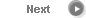#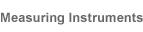# Precise Color Communication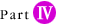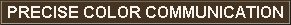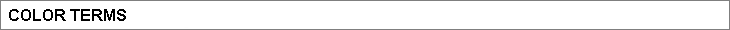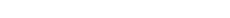Color difference ∆E*ab in the L*a*b* color space, which indicates the degree of color difference but not the direction, is defined by the following equation: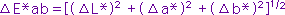where ∆L*, ∆a*, ∆b*: Difference in L*, a*, and b* values between the specimen color and the target color.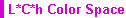The L*C*h color space uses the same diagram as the L*a*b* color space, but uses cylindrical coordinates. Lightness L* is the same as L* in the L*a*b* color space; Metric Chroma C* and Metric Hue Angle h are defined by the following formulas: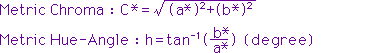where a*, b*: Chromaticity coordinates in the L*a*b* color space For difference measurements, Metric Hue Angle difference is not calculated; instead, Metric Hue Difference ∆H* is calculated according to the following formula: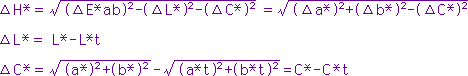The Metric Hue Difference is positive if the Metric Hue Angle h of the specimen is greater than that of the target and negative if the Metric Hue Angle of the specimen is less than that of the target.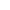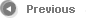5/12